Math Courses / Course / Chapter

# Algebra Symbols, Meanings & Chart

Brigette Banaszak, Ellen Manchester
• Author
Brigette Banaszak

Brigette has a BS in Elementary Education and an MS in Gifted and Talented Education, both from the University of Wisconsin. She has taught math in both elementary and middle school, and is certified to teach grades K-8.

• Instructor
Ellen Manchester
Learn about algebra symbols and their meanings. Understand different symbols such as the inequality symbols and their meanings and find an algebra symbols chart. Updated: 11/23/2021

Show

#### What is the most popular symbol in algebra?

The most widely used symbol in algebra is the variable. A variable is a symbol, usually a letter such as x or y, used to stand for an unknown quantity.

#### What are the symbols in algebra?

There are a wide variety of symbols in algebra, ranging from variables (x), the equal sign (=), and the less than sign (<). These symbols are used as a quick and easy way to show a meaning that mathematicians everywhere will understand.

#### What are the basic math symbols?

Basic math symbols include addition (+), subtraction (-), multiplication (x), and division (/). Other common math symbols are the equal sign (=), the less than sign (>), and the greater than sign (>).

What are algebra symbols and signs? Algebra symbols and signs are often used in mathematical equations. An algebra symbol or sign has a consistent, understood meaning. Mathematicians in any country, speaking any language, will understand and agree upon the meaning of the symbol. These symbols bring consistency to mathematical expressions and equations. Algebra symbols and signs are also a shorter, easier way to write, read, and do computations. Trying to write out the meanings of the various symbols in words would be a waste of time and energy.

There are a variety of different algebra symbols and signs used. Understanding different algebra symbols and their meanings will help in performing and understanding computations.

One well-known algebra symbol is the variable. But, what is a variable? A variable is a symbol, usually a letter, that stands for an unknown quantity. Letters are generally used, particularly the lowercase x and y, but a variable can be another symbol, such as a box. In the equation {eq}5n\ -\ 17\ =\ -250 {/eq}, the letter n is a variable standing for some unknown quantity.

### Equality Symbols

What are equality symbols and their meanings? The best-known example of equality is the simple equal sign, {eq}= {/eq}, which means the same as. It is used to show the expressions on either side have the same value. For example, in the equation {eq}8\ +\ 7\ =\ 15 {/eq}, the two sides of the equation both have a value of 15.

There are other equality signs as well, however. The symbol {eq}\approx {/eq} means approximately equal. This symbol is used to show the two sides of the equation are approximately or about equal, such as {eq}\frac{2}{11}\ \approx\ 0.22 {/eq}.

Another equality symbol is the congruent symbol, which is {eq}\cong {/eq}. This symbol can be used in geometry to show geometric shapes have the same shape and size. Another equality symbol used in geometry is the similar symbol, which is {eq}\sim {/eq}. This symbol is used to show geometric shapes have the same shape but different sizes. The diagram shows three squares.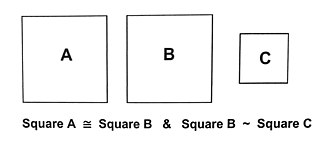Squares A and B have the same shape and size, so they are congruent, represented through the symbol {eq}\cong {/eq}. Squares B and C have the same shape but different sizes, so they are similar, represented through the symbol {eq}\sim {/eq}.

The final equality symbol to be familiar with is the not equal symbol, which is {eq}\neq {/eq}. This means two sides of an equation are not equal, such as {eq}5\ +\ 3\ \neq\ -8 {/eq}.

To unlock this lesson you must be a Study.com Member.

## Variables = Letters = Unknowns

In algebra, a variable is the use of a letter, usually x and y, to represent an unknown quantity. For instance, if you have 7 cookies, and you're left with 3 after your friends leave, algebra and the use of a variable can help you find out how many were eaten: 7 - x = 3, or x + 3 = 7.

Typically, the first symbol in an algebra problem is the letter, or variable. However, either form of the equation shown above can be used to translate a word problem into an algebraic equation.

Algebra symbols and signs are used in mathematical equations. An algebra symbol or sign has a consistent, understood meaning. Mathematicians in any country, speaking any language, will all understand and agree upon the meaning of the symbol. These symbols bring consistency to mathematical expressions and equations.

Here are some of the better-known algebra symbols.

• A variable is a symbol, usually a letter such as x or y, that stands for an unknown quantity.
• The equal sign is {eq}= {/eq}.

To unlock this lesson you must be a Study.com Member.

## Symbols of Equality

Most of you are probably familiar with basic equals sign, which means 'the same as': =.

For instance, 3x - 2 = 4 means three times an unknown minus 2 is the same as 4.

The equal sign is important because it forms the balancing point or fulcrum of the equation. Whatever mathematical operation you perform on side of the equation, you must also perform on the other side.

In solving algebraic equations, you'll also come across an altered equal sign with three bars: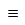In algebra, an altered equivalence symbol means: 'is identical to' or 'is equal by definition'. Another symbol that indicates a number or quantity is almost equal to another number or quantity is: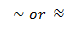As used here, the squiggly lines indicate that the numbers on either side of the equal sign are close to, but not exactly the same as, each other. So, if you wanted to find the value of the square root of 2 = 1.41421356…, you'd say the square root of 2 is about 1.4. When working with approximations, this equivalence symbol is the best one to use.

## Symbols of Inequality

We use inequality symbols when the solution has a range of answers. For instance, the difference between x = 4 and x > 4, is that x > 4 indicates all the numbers larger than 4 through infinity, while x = 4 only refers to the number 4.

Inequality symbols are read from left to right. When the smaller side appears first, it's the less than symbol. When the larger side appears first, it's the greater than symbol. For example, you'd use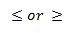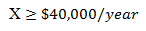Let's look at another example, if you owned a business and wanted to work no more than 50 hours per week, you'd use the 'less than or equal to' symbol and write it this way: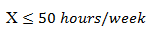## Symbols of Organization

Parenthesis, brackets and braces help to keep algebraic equations organized and show which operation to perform first. Remember that, in algebra, we begin solving problems from the inside out, staring with the parentheses and then moving onto the brackets and braces. Let's use the order of operations to simplify this equation:

3{5(2-3) (4+1) - (6-7)} + 3: First, perform the calculations shown in parentheses.

3{5(-1) (5) - (-1)} + 3: Multiply the numbers inside the brackets or 5(-1).

3{5-5+1} + 3: Add the numbers inside the brackets or 5 + 1.

3{5[-4]} + 3: Multiply the numbers inside the braces or 5*(-4).

3{-20} + 3: Multiply 3 and -20.

-57: Solution

## Variables = Letters = Unknowns

In algebra, a variable is the use of a letter, usually x and y, to represent an unknown quantity. For instance, if you have 7 cookies, and you're left with 3 after your friends leave, algebra and the use of a variable can help you find out how many were eaten: 7 - x = 3, or x + 3 = 7.

Typically, the first symbol in an algebra problem is the letter, or variable. However, either form of the equation shown above can be used to translate a word problem into an algebraic equation.

## Symbols of Equality

Most of you are probably familiar with basic equals sign, which means 'the same as': =.

For instance, 3x - 2 = 4 means three times an unknown minus 2 is the same as 4.

The equal sign is important because it forms the balancing point or fulcrum of the equation. Whatever mathematical operation you perform on side of the equation, you must also perform on the other side.

In solving algebraic equations, you'll also come across an altered equal sign with three bars:In algebra, an altered equivalence symbol means: 'is identical to' or 'is equal by definition'. Another symbol that indicates a number or quantity is almost equal to another number or quantity is:As used here, the squiggly lines indicate that the numbers on either side of the equal sign are close to, but not exactly the same as, each other. So, if you wanted to find the value of the square root of 2 = 1.41421356…, you'd say the square root of 2 is about 1.4. When working with approximations, this equivalence symbol is the best one to use.

## Symbols of Inequality

We use inequality symbols when the solution has a range of answers. For instance, the difference between x = 4 and x > 4, is that x > 4 indicates all the numbers larger than 4 through infinity, while x = 4 only refers to the number 4.

Inequality symbols are read from left to right. When the smaller side appears first, it's the less than symbol. When the larger side appears first, it's the greater than symbol. For example, you'd useLet's look at another example, if you owned a business and wanted to work no more than 50 hours per week, you'd use the 'less than or equal to' symbol and write it this way:## Symbols of Organization

Parenthesis, brackets and braces help to keep algebraic equations organized and show which operation to perform first. Remember that, in algebra, we begin solving problems from the inside out, staring with the parentheses and then moving onto the brackets and braces. Let's use the order of operations to simplify this equation:

3{5(2-3) (4+1) - (6-7)} + 3: First, perform the calculations shown in parentheses.

3{5(-1) (5) - (-1)} + 3: Multiply the numbers inside the brackets or 5(-1).

3{5-5+1} + 3: Add the numbers inside the brackets or 5 + 1.

3{5[-4]} + 3: Multiply the numbers inside the braces or 5*(-4).

3{-20} + 3: Multiply 3 and -20.

-57: Solution

To unlock this lesson you must be a Study.com Member.

### Register to view this lesson

Are you a student or a teacher?

Back

### Resources created by teachers for teachers

Over 30,000 video lessons & teaching resources‐all in one place.Video lessonsQuizzes & WorksheetsClassroom IntegrationLesson Plans

I would definitely recommend Study.com to my colleagues. It’s like a teacher waved a magic wand and did the work for me. I feel like it’s a lifeline.

Jennifer B.
TeacherCreate an account to start this course today
Used by over 30 million students worldwide